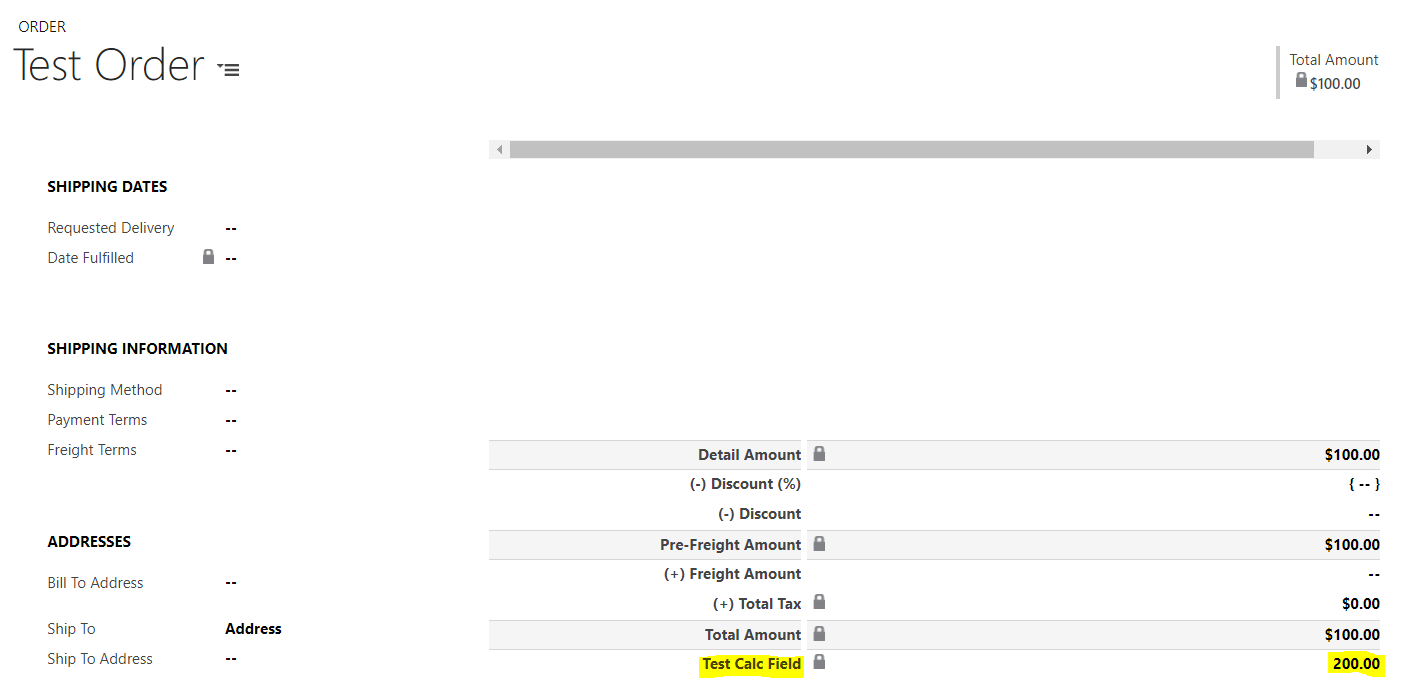# Dynamics 365 Calculated Fields

Dynamics 365 has built in functionality for calculated fields. These are fields that can be set up without code. Here we will go through an example.

Let’s assume we need a new calculated field on the Order entity. Let’s create a new field. Enter the display name and select the data type. Now select the field type. You can see here there are the options:

• Simple
• Calculated
• Rollup

Note, not all of these field types are available for all data types.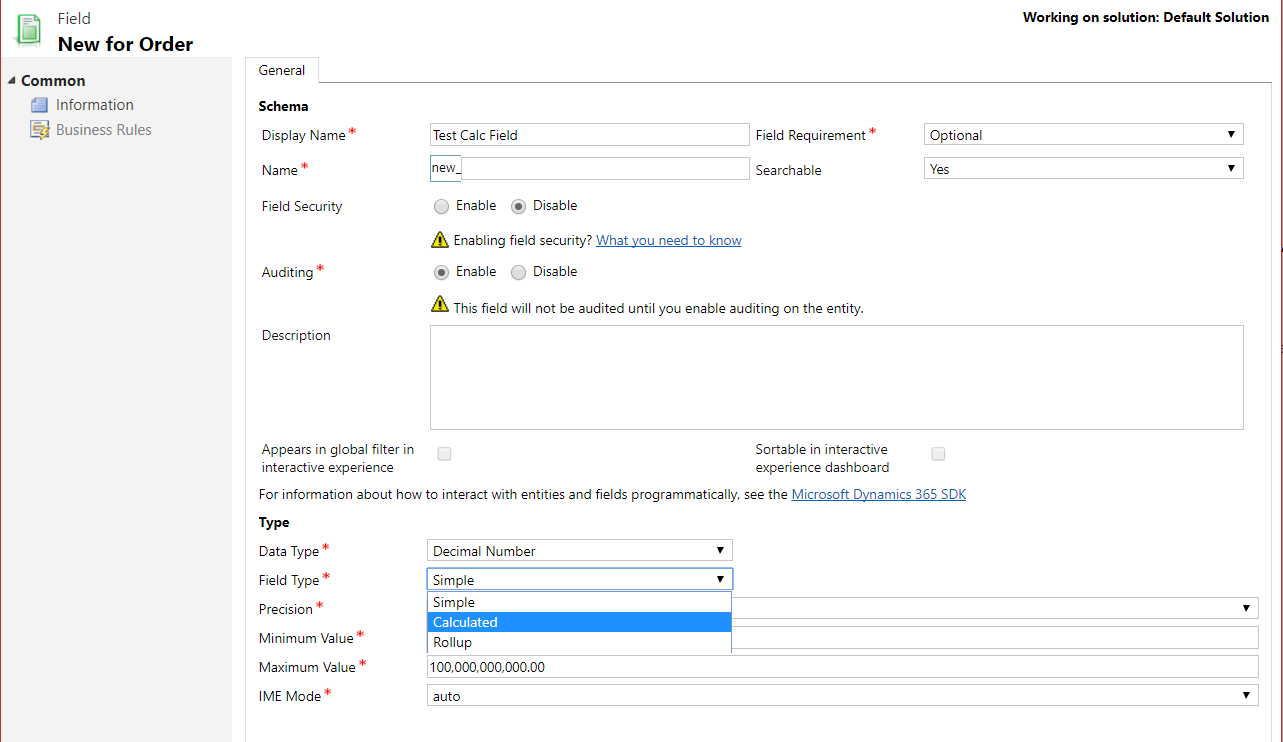Select Calculated. We now see an Edit button.You will see the calculation window open: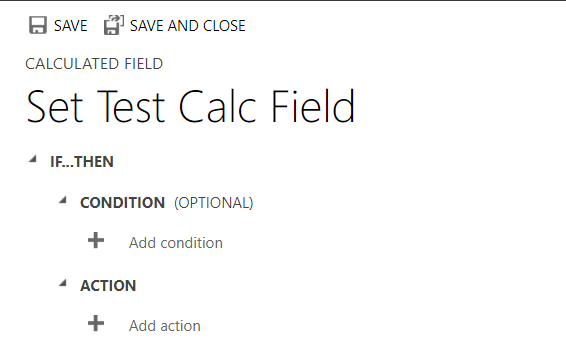You can add a condition, which is optional: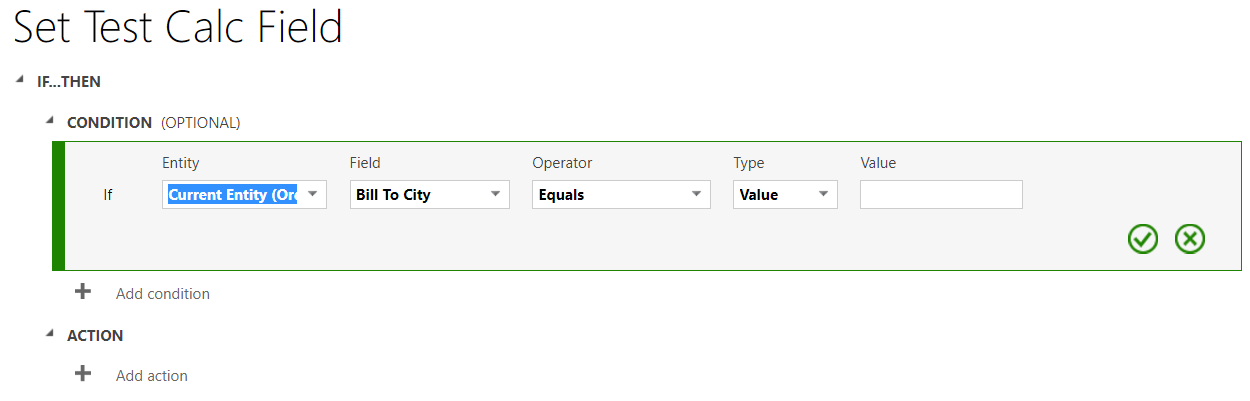And an Action: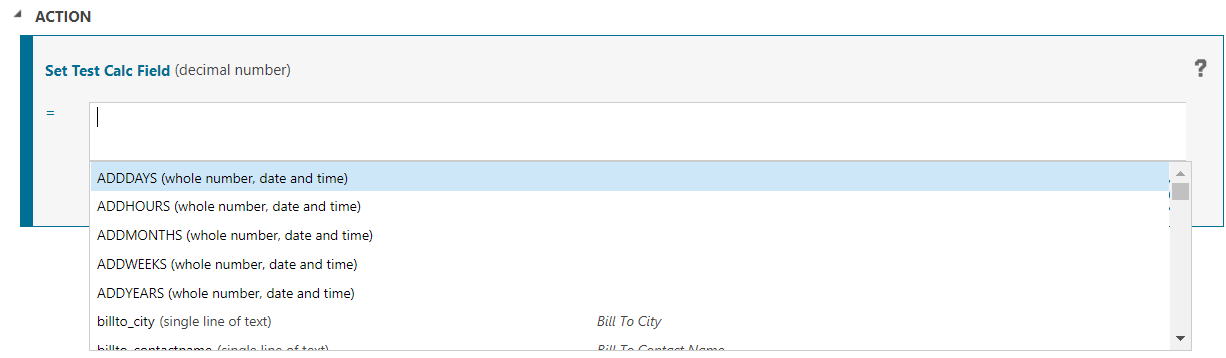As a simple example, let’s add the total amount to itself: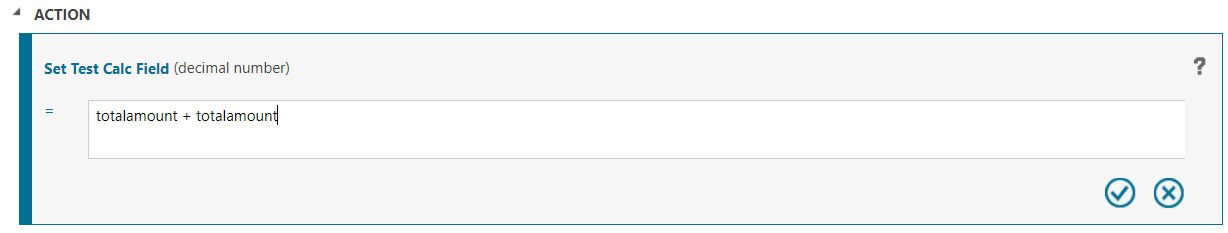Save the field and open the form. We will add the field to the form: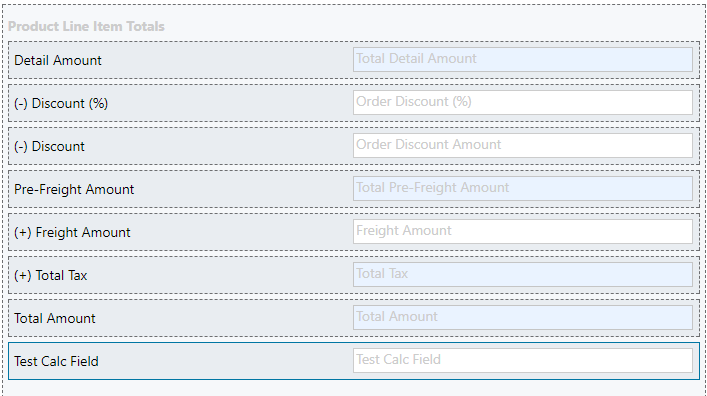Save and publish the form.

Displaying a previous order, you will see the new calculated field and its value: